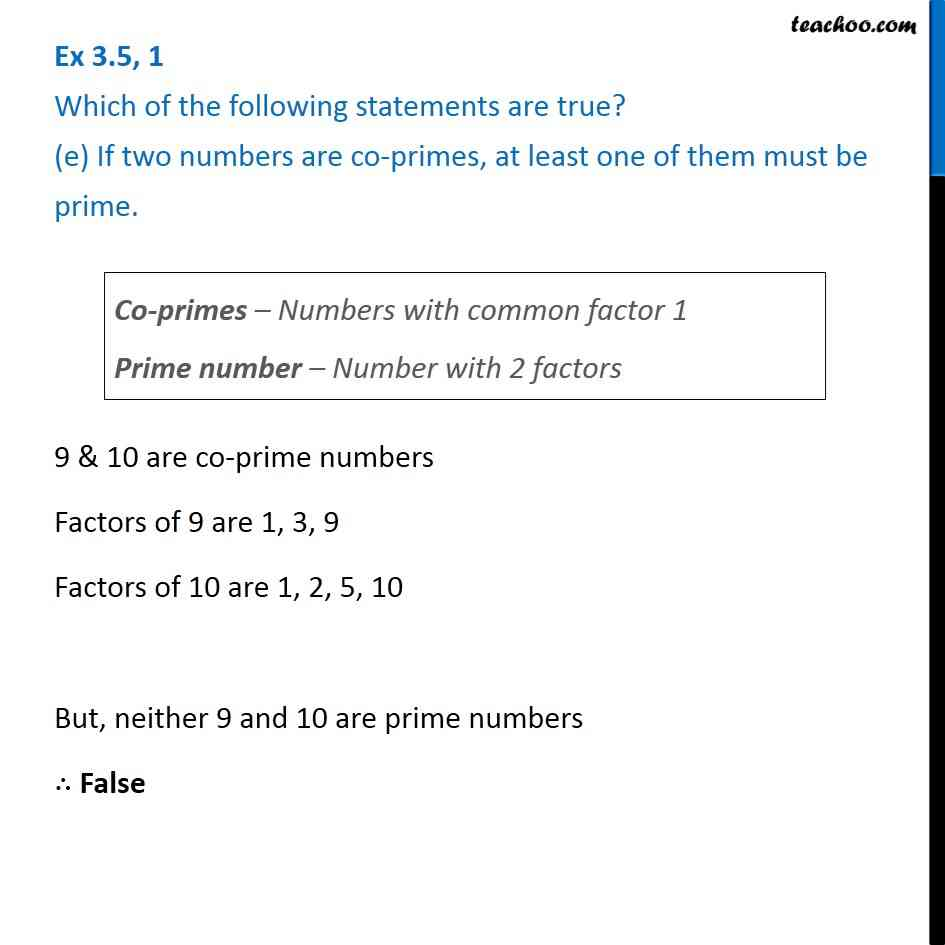1. Chapter 3 Class 6 Playing with Numbers
2. Serial order wise
3. Ex 3.5

Transcript

Ex 3.5, 1 Which of the following statements are true? (e) If two numbers are co-primes, at least one of them must be prime.Co-primes – Numbers with common factor 1 Prime number – Number with 2 factors 9 & 10 are co-prime numbers Factors of 9 are 1, 3, 9 Factors of 10 are 1, 2, 5, 10 But, neither 9 and 10 are prime numbers ∴ False